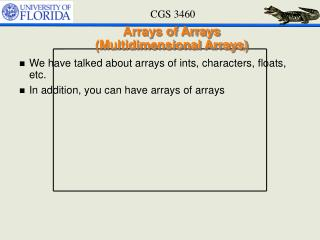DownloadDownload PresentationArrays of Arrays (Multidimensional Arrays)

# Arrays of Arrays (Multidimensional Arrays)

Download Presentation## Arrays of Arrays (Multidimensional Arrays)

- - - - - - - - - - - - - - - - - - - - - - - - - - - E N D - - - - - - - - - - - - - - - - - - - - - - - - - - -
##### Presentation Transcript

1. Arrays of Arrays(Multidimensional Arrays) • We have talked about arrays of ints, characters, floats, etc. • In addition, you can have arrays of arrays

2. Multidimensional Arrays • Declaration • type name[ mrows][ncols ]; • e.g. int matrix; • What it means? • Declare an array of size mrows • Each element of that array will be another array of size ncols of the given typ • Access • matrix; • Not matrix[3,4], or matrix(3)(4) m–by–n matrix j changes n columns m rows i changes

3. Multidimensional Arrays • Initialization • int M = {{1, 2, 3}, {4, 5,6}}; M M

4. Multidimensional Arrays • int M = {1, 2, 3, 4, 5, 6}; • Numbers will fill up the first row, then the second row,… M M

5. Multidimensional Arrays • int M = { = 1,=3}; M M

6. Arrays and Pointers • Recall arrays are pointers • int a; • a is a pointer to a location in memory where space for 10 ints is reserved • int b • b is an array of arrays • b gives and array of ints of size 20 • Since arrays are pointers • b is also an array of pointers • b can also be considered as a pointer to a location in memory where space for 5 integer pointers is reserved • b is a pointer to a pointer to an integer

7. Dynamic Allocation of 2D arrays • Remember malloc • malloc(sizeof(int) * 15) would reserve space for 15 ints and return the address of the first int • What would malloc(sizeof(int *) * 10) do? • reserve space for 15 integer pointers and return the address of the first int

8. Dynamic allocation of 2 D Arrays • Want to declare an integer array with M rows and N columns called A. • What is A’s type? • int ** A; • What should A point to? • Array of integer pointers (int *) • A = malloc(sizeof(int*) * M) • A[i] is a different pointer to an int for every I • What should A[i] point to? • Array of integers (int) • A[i] = malloc(sizeof(int) * N) for all i • A[i][j] gives you an int at the i, j index

9. Summary int** A; int M, N, i, j; A = malloc(sizeof(int*) * M); for(i = 0; i < M; i++) { A[i] = malloc(sizeof(int) * N); }

10. Two dimensional character arrays • char A • Contains 5 arrays of 100 characters • Could contain 5 arrays of strings (with max size 99) • A  “foo” • A  “bar” • etc.

11. Command line arguments • Command-line arguments • Given after the name of a program in command-line • cp f1 f2 • gcc yourfilename • Arguments are passed in to the program from the operating system • Flexible • But not required

12. How to Interpret • A command-line interpreter explains the command • The first word is treated as the name of a program • Others as arguments. • These strings are past to main function in C cp f1 f2 NULL cp f1 f2

13. How to Declare • In C, these strings are past to the main function • main() can actually accept two arguments • number of command line arguments • a full list of all of the command line arguments. • Declaration • int main ( int argc, char *argv[] )

14. How to use • argc • Argument count • Number of strings – including executable file name • argv • Argument vector • Size of argc+1 cp f1 f2 NULL cp f1 f2